# Find the Fish – Penrose Fish

 Try to match the fish to the pattern so that it fits completely. How many fish can you find? What is their pattern? How many different shapes make up the poster pattern? The poster uses seven different configurations around a point. Can you find them all? Are any possibilities missing?
Maths

Covering a flat surface with geometric shapes is called a tiling. For example, a floor might be tiled with squares. We can also use more than one shape, here is a tiling that uses two shapes: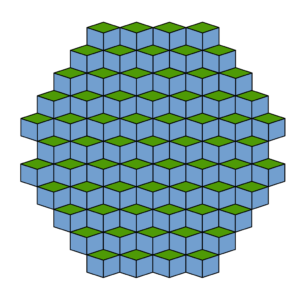This pattern continues infinitely in all directions. Notice how the pattern repeats itself – in other words, shifting all the tiles across gives the exact same pattern. This is called periodic.

The pattern below is made from two geometric shapes, but this time the pattern does not repeat itself – shifting the tiles across never produces the exact same pattern.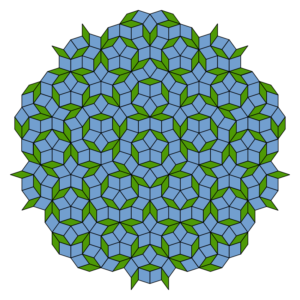In fact, there are many ways to tile a surface using these two shapes, but the tilings will never be periodic. So, we call the tilings aperiodic.

The poster is an example of the simplest kind of aperiodic tilings, known as Penrose tilings. The Penrose Fish tiles are an example of a different Penrose tiling.

Parts of a Penrose tiling have the same symmetry as a pentagon (five-fold rotational symmetry and five mirror lines of reflective symmetry). This only true for finite areas (not the whole infinite tiling), but the finite areas can be quite large.

History

Tilings have been used as decorative art since ancient times, from Islamic art to Roman mosaics. Tilings can be made from one or more shapes, which might be regular or irregular polygons. If the pattern repeats the tiling is called periodic.
In 1966, mathematician Hao Wang and his student Robert Berger, found a set of colourful square tiles that were not periodic (aperiodic). This idea was adapted by mathematician Raphael Robinson to create aperiodic tilings using six shapes.
In 1974, British mathematician Roger Penrose discovered new aperiodic tilings that used six shapes, and two shapes. These tilings were based on pentagons rather than squares.

PeopleHao Wang 1921- 1995 Hao Wang was a Chinese mathematician and philosopher who later worked at Oxford University and Harvard University. In 1959, Wang wrote a computer program for proving mathematical theorems. In 1966, Wang and his student Robert Berger found a colourful set of square tiles, which are the first noted example of aperiodic tilings.Roger Penrose 1931 – Present Roger Penrose is an English mathematical physicist at the University of Oxford. Penrose’s work on the mathematics of spacetime lead to the theoretical existence of black holes. Penrose also discovered ways to tile a surface with two shapes in a pattern that never repeats, known as Penrose tilings.
Applications

Aperiodic tilings were only discovered by mathematicians in the 1960s. Since then they have been used as art and as decoration in architecture, including the floor of the department of mathematics at Oxford University and at San Francisco’s transit centre.A quasicrystal is a material with aperiodic structure. Although the mathematics of quasicrystals has been known for some time, the first naturally occuring quasicrystal was found in 2009, it is an alloy of aluminium, copper and iron called icosahedrite. Quasicrystals have unique material properties and applications are being investigated.

Maths at Home

Print and cut out these tiles to make a pattern.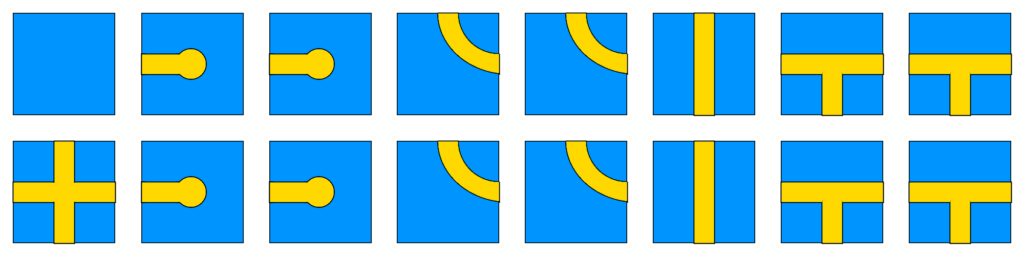The tiles are called Wang tiles. If the pattern doesn’t repeat it is non-periodic.

Here is another set of Wang tiles discovered in 2011. For this set, the patterns will never be periodic.

The most famous examples of aperiodic tilings are Penrose tilings. One set uses two tiles, called kite and dart. The idea here is to match the lines, so although it’s tempting, we cannot use the kite and dart to make a rhombus.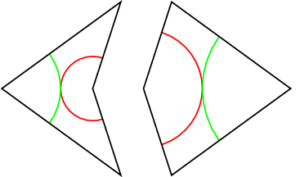Download and print your own set of tiles here. This is the set of tiles found on the Find the Fish poster.

Another set of Penrose tiles uses a thin rhombus and a fat rhombus.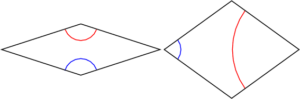Download and print these tiles here. This is the set of tiles used in the Penrose Fish activity.